# The Computer

## Computing

A certain club had several procedures, which ran as daily decisions made by its managers They were numbered, properly described and stored.
On that day, George, the door man, received instructions to perform the procedure number 5 during the opening hours and to perform the procedure number 8 after closing and before leaving.
Then George fetched the procedure No. 5, which consisted on allowing the entry to:

1. People with 18 years old and over,
2. People with a prepaid entry receipt,

And only if these two conditions were met, customers got in after George had registered their sex and age.
He read and put the procedure No. 5 in his memory, so that he could execute it. As he was easily distracted and failed systematically some steps in the process, he arranged a simple way to ensure that all steps were always fulfilled: he built a switch system linked as complied with the procedural steps. Only after they were all connected, it lit a light that warned that he could let the customer in.
When the night club closed, George went to the storage room, put the procedure number 5 and collected the procedure number 8, who he read and memorized. This procedure said that he should, with the registered data, determine the client’s percentage by gender, calculate their average age and determine the number of customers entered that day. It should then store those records and leave a report with the process’s result on the manager’s table, so that with this information, he could make decisions regarding the club’s management.
After this done, he went home.
George collected client’s data, performed procedures or programs with those and obtained information that enabled decision making.
Let’s make some analogy between George and a computer.

He went to the storage room (HDD) and got a procedure (Program) that placed into his memory (MP) so that he could execute the instructions of the procedure (Processing)  with the elements that he would collect from customers (Data),  and with the results obtained (Information) to allow customers to get in (Decision).

He executed a conditional operation: if, after fulfilling all instructions, all necessary conditions would be verified, he would let the customers get in. And he made ​​it as a computer, by turning on and off switches.
We can see the analogy between a machine and the human behavior, for instance in a parking lot. The machine verifies the presence of the car (through volume detectors), the parking’s payment (through the introduction of the payment card) and communicates the data to the computer which, in return connects or not the two switches , the condition that allows to close or not the circuitry which raises the barrier.
This chapter’s introduction intends to allow a better knowledge of the four key elements in a computing operation:

1. Data, corresponding to the specific characteristics of what is intended to process;
2. Data processing corresponding to the execution of a program;
3. Information obtained through data processing;
4. Decision that can be held based on the knowledge provided by such information.

Processing is the execution of a process. And a process is the way, the method and the sequence we use to do certain things. To this kind of process, when inside a computer it’s called program and to its execution processing.

• Under Procedure No. 5, the process corresponds to a simple conditional logic operation’s execution.
• Under Procedure No. 8 the process corresponds to a mathematical calculation, which translated in terms of computer language, is the implementation of a far more complex operation of mathematical logic.

Yes, it’s good to remember that the computer can only understand on and off situations. It’s a machine of switches. To understand how this machine of switches works, we need to get down to its nature and way of operating. Understand how we shall talk to it it’s our purpose.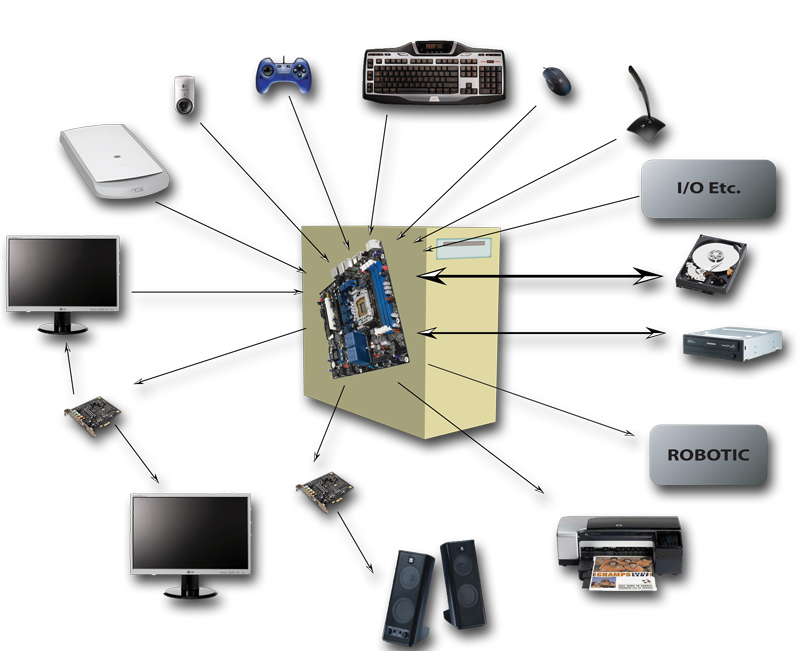## How do we intercommunicate

The most common forms of interactive communication with the computer are the keyboard, the mouse and the monitor.
It’s through the monitor that the computer asks for the data it needs for processing, and subsequently give us the information. For this purpose it uses a Graphical User Interface (GUI). This is the fancy name for the ordinary windows that work on the monitor, which are attractive and helpful workspaces with suitable locations for data entry and options selection.
It’s by clicking these options with the mouse and filling the due spaces with the keyboard that we provide the computer with the data  to be processed, whose result (the information) will be  returned to us  also through the monitor.
But there are other forms of communication, which provide the computer the data it expects. Be the case for optical drives, volume detectors, photoelectric cells , tactile displays, drawing boards, photographic or video cameras, scanners, microphones, gaming devices and all those who already exist or the human imagination will create.

There are many other ways the computer uses to provide us the information, that we will not enumerate and whose paths are almost identical to those of the printer or the monitor. Here it’s important to refer the means in which the computer communicates by running actions, i.e., deciding based on information, as shown in the park gate opening case, in which it did or not close the electrical circuit responsible for opening the park gate. A simple example that shows the path for robotization and the increasing automation of computer-assisted production.

Concerning the connection between the outside world and the computer and vice versa, we have been able to define what is called I/O from “Input/Output Devices”.

## How the Computer Arose

Now that we’ve made a small approach on what the computer does and how it communicates with us and we with him, let’s try to understand what caused its birth.
Many years ago, more than a century, a gentleman named George Boole determined that it was possible through the laws of thought to create expressions that would solve all algebra problems through logic. It was the true/false duality usually noted as 1/0 respectively, replacing the usual numeric variables, and the operations of addition and multiplication replaced by the logical conjunction (AND), disjunction (OR) and negation (NOT).
How could this man imagine what a century later would arise following the establishment of its algebra and propositional logic. And all because another gentleman named Claude Shannon insisted and showed that it was possible to recreate the mathematical abstraction of Boolean algebra through electrical circuits and switches (large relays) that he would call and hang up in order to form the necessary circuits. But this reality we will approach latter.
Now we must retain the assertion ago produced that the Boolean algebra replaced numerical variables of traditional Algebra for true/false duality, usually noted as 1 and 0. Thus, 1 and 0 are the digits required to solve all the expressions of Propositional Logic and consequently all Mathematical problems. So this would be the language of the machine, since as we said the computer was created to run the expressions of Propositional Logic

# The language of the Computer

## Its vocabulary

The computer can be seen as a machine of switches. It only knows how to identify the on or off situations present in its circuits and doesn’t recognize any other situations, and as it goes turning on and off switches (transistors), it causes active or not active outputs in the circuits in which they are inserted, it charges or doesn’t charge capacitors, it introduces the value on/off  in the form of with/without voltage in other circuits, sequentially increasing the complexity of the combinations. But no matter how big the complexity is, we must never forget that it comes from situations based in on/off in  the form of with or without voltage, charged or empty. Resuming, we’ll always have only two possible options.
Before we go any further with the analysis of real  and material implementation of what we know to be a computer, with these basic concepts, we’ll take a detour through the  field of abstraction of logical and mathematical human reasoning.
In fact, the duality concept represented by the absolute power on/off corresponds to the duality of logical true/false as the value of a proposition or statement, to which we assign the corresponding value of “1 or 0“. This is the only vocabulary we have, to talk with the computer. Two digits: 1 and 0. Two digits which turned the creator dependent on the creation.
In order to proceed, let’s do a short introduction to the concept of positional numeral systems, in which is included the one we humans use:  the decimal numeral system or base 10 number system.
The base, in the numeral system, represents the number of digits available to represent a number. In Base 10 there are 10 digits (0 to 9).

## Positional Numeral System

Taking these elemental concepts:

• A number, is a way to represent an amount of anything.
• A digit is a one-digit number that ranges from 0 up to a value prior to the one of the base to which it belongs (0 to 9 in base 10 – 10 digits).
• A numeral system is a systematic way to represent numbers.

We can define the positional numeral system as a systematic way to represent numbers based on the value of each digit (always lower than the base) and its position in the representation of the number (set of digits).
Understood? Hmm ..! Better we go otherwise.

### Decimal Numeral SystemIt’s assumed that the decimal or base 10 numeral system was adopted by man in early times due to its equivalence with the number of fingers. Indeed, even nowadays it’s common to see people counting by their fingers.
In base 10 positional numeral system, each digit represents the value of its multiplication by the base value, as many times as its position in the number’s representation from right to left or, by the value of the base raised to the power of its position. The first position is set to 0 (any number raised to the power of 0 is equal to 1). The value of the number intended to be represented, results from the sum of all these plots, as we can see in the table here represented.
Take for instance the number represented by the digits 491622. Multiply each digit by the value of the base as many times as its position in the number’s representation, counting from right to left, with the first digit set to position 0. Then sum all the plots. We’ll get 491,622.
Since we can represent one number in any base, we will now analyze the base 16 and see how it works.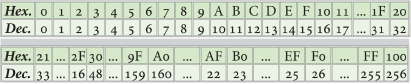Hexadecimal numeral system, or base 16 positional number system, needs 16 digit to represent a number.
Because, in addition to 9, there are no simple numerals to represent the base digit values (in our decimal concept figure) alphanumeric symbols were selected (A, B, C, D, E, F) to represent the digits missing . Thus, hexadecimal digits are as shown in table, by analogy to its value in the decimal system.In any base other than our decimal base, the set of numbers 10 should be read not ten but “one, zero” and so on.
Let us now see what’s the decimal value which corresponds to the hexadecimal value of FF, like we do in Figure 1-4a, using the same procedure as in base 10.
This means that in hexadecimal, two digits can represent 256 values ​​(from 0 to 255) in decimal.

### Binary Numeral System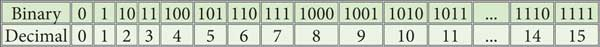The binary numeral system corresponds exactly to the vocabulary of the Computer. As the name implies it is composed of two digits: 0 and 1. Remember that we are in base 2, which means we need 2 digits to represent any number, as we can see in the table together  representing a 4 digit number in base 2 and its equivalence in base 10.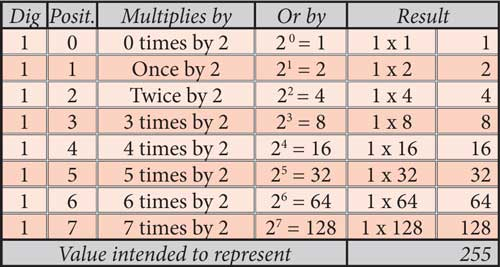Now we will see what is the value that corresponds in base 10 to the number represented by 11111111 in base 2 as represented in Figure 1-5a. Look! It’s exactly the same value as FF in base 16.
The decimal value represented by FF in base 16 is the same represented by 11111111 in base 2.
One 8 digit number in base 2 is represented by one two digit number in base 16. Not one, but all the possible numbers in both combinations.
This makes the representation of binary compositions much easier, even better than in the decimal system.
For this reason, the hexadecimal system is usually used in computing to represent the structure of the binary machine language.
Computers use sets of 8 numbers 0 or 1 (the Byte), as the basic unit of addressing and operation. All possible combinations of 8 digits can be represented by two hexadecimal digits, also depleted in all combinations.
As we have seen before, the vocabulary of the computer is reduced to the numbers 1 and 0,  which represent the abstraction true or false, becoming evident that the binary numeral is the ideal set of digits to talk with it . Hence the importance of this numeral system in computer science.
Once found the formula for man and machine to communicate each other, the first through two-digit numeral system and the second through switches that it turns on and off, let’s move on to a fundamental concept, which is the basic unit of information and its multiples.

# The Information Units

## Bit (“BInary digiT”)

Bit is the smallest unit of information that can be stored or transmitted.
Physically, the value of a bit is generally stored as an electrical charge below or above a standard level in a single capacitor in a memory device. However, bits can be represented physically by several means. The means and techniques commonly used are:

• By electricity, as already mentioned,
• By way of light (fiber optics and optical disk readers or writers for example),
• Via electromagnetic waves (wireless network), or
• By means of magnetic polarization (hard disks).

But you can not do much using only one bit alone, as we would not understand each other using single letters.

## ASCII Code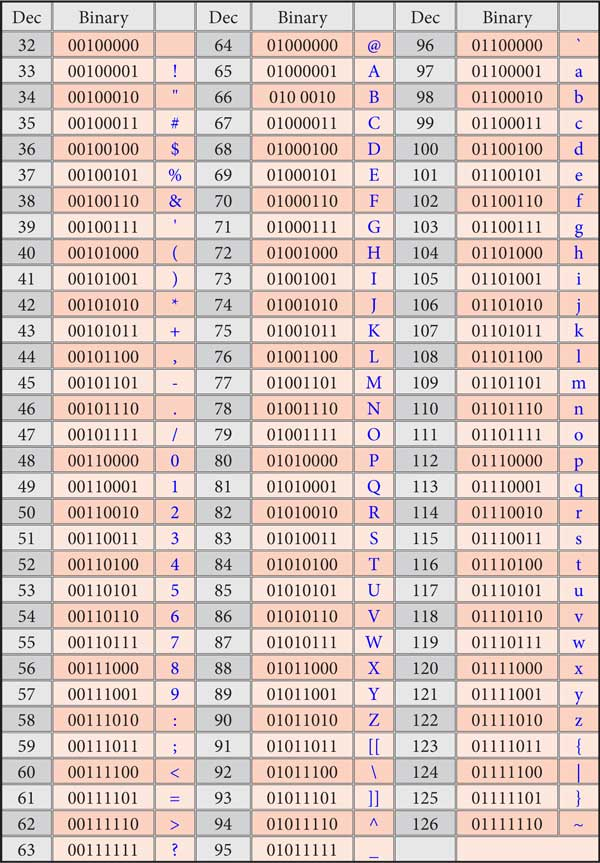As the computer only understands numbers, namely 0 and 1, the first concern of the developers was to find a code representative of all the letters of English alphabet, numbers, basic keyboard commands, punctuation and special symbols.
It would be that way that the computer, relating certain graphic symbols with its representative binary code, could be meaningful for us.
Thus, they proposed a code originally accepted in America and quickly spread to the whole world, which is called ASCII (American Standard Code for Information Interchange which translates as “American Standard Code for Information Exchange”), representing 128 possible characters, by a combination of seven bits.
The ASCII code had the following representation:

• Codes 0-31, non-printable characters, were control characters representing actions usually contained on the keyboard,
• Codes 65-90, representing the uppercase letters,
• Codes 97-122 representing the lowercase letters and
• The remaining Codes, representing numbers, punctuation and various symbols,

as we can see in the table of ASCII printable characters here together.
The ASCII code was conceived for the English language. So it did not contain accented characters or special characters from other European languages. To encode this type of characters and extend ASCII to Europe, it was necessary to use additional encoding. The ASCII code was then extended to 8 bits, in order to be able to encode more than 128 characters, being called the extended ASCII code.
This extended ASCII code uses 256 possible combinations, ranging from 00000000 (decimal 0) to 11111111 (decimal 255), therefore being codified in combinations of sets of 8 bits.

## Byte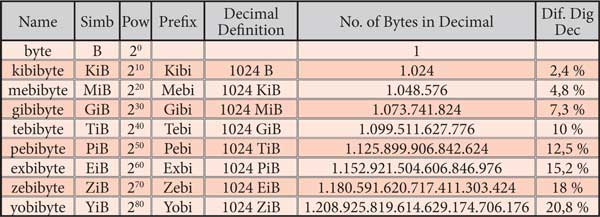One Byte has nothing special, beside being a set of 8 bits. Its importance in computing derived solely from the fact that the extended ASCII code has adopted sets of eight bits to represent in the language of the computer the characters and actions that we understand, thus making this set of bits the standard form of communication of a computer.
It was from this fact that the Byte concept arose, corresponding to a set of 8 bits which, due to its hard use, became the standard unit of information. Thus, the coding standard byte is set to be 8 bits.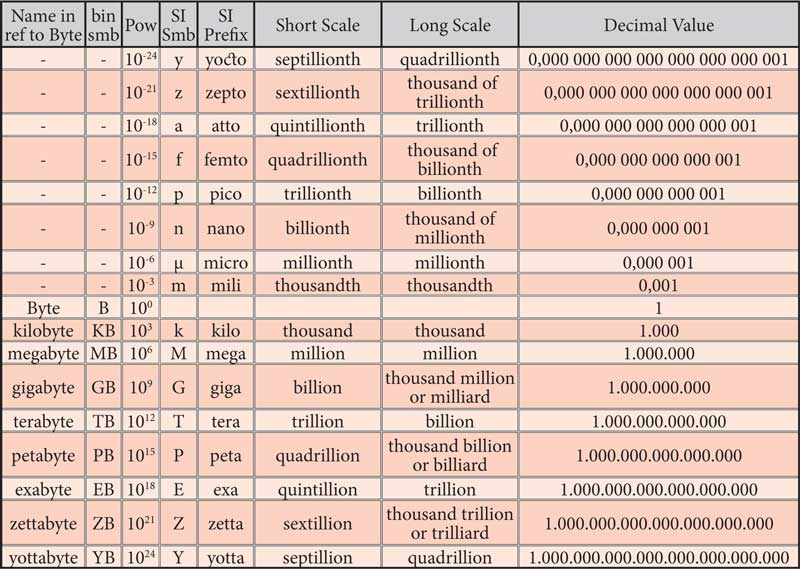The storage capacity of memory devices and data storage devices, is usually referred to as a multiple of Byte. The Byte is the smallest unit that the CPU addresses. With regard to the memory, whatever the size of the word, the addressing is always made to the Byte.
Typically, the SI prefixes are used to designate powers of Bytes and are understood by those who hear them as if they were powers of 10. But is not the case and the percentual difference, as you can see in the most right column of the table in Figure 1-7, can reach very significant values​​.
When referring one HD with 1 terabyte, actually it has about 10% less capacity than that it should have, since that reference is understood as made in binary numeral system.
In the case of HD, when referring multiple of bytes, consequently implying the binary numeral system, their manufacturers are actually producing them as multiples of 10.
Just for the sake of curiosity and completion of the information, we add the tables  describing the values regulating the systems of units, in binary and decimal numeral systems.

## Word

Word, is the natural unit of information used by a specific CPU type. Is a group of bits of fixed size, which is processed together.
The word began as a Byte, or 8 bit (8 bit processors), then evolved into 16 bit (16 bit processors), recently passed to 32 bit (32 bit processors) and is now becoming common with 64 bit (64 bit processors).
The number of bits of a word (word length) is an important feature of a computers architecture. It is reflected in many aspects of the computer’s arquitecture, which will have the width of the word:

• The Registers, which are specific memories of the CPU where the data being processed is placed,
• The Program Counter, which is a register pointing to the next program instruction to be executed,
• The data and the address buses

will all have the width of the word.
Although it doesn’t have to be multiple of the byte, these are the most common sizes of the word.

# Conclusion

We verified that base 2  or binary  numeral system, is perfect for our conversations with the computer, because it represents its absolute duality in states of voltage or no voltage.
We verified that the basic unit of information containing one of the two possible digits of base 2 is the bit and we have previously spoken of the enormity of those stuff which can be stored and worked on simultaneously .
Now it seems we’re listening to the pertinent question:
And what are we going to do with all this vermin?”
It’s now time to move on and address the issue of Logical Mathematics and Boolean Algebra, to understand how this small vocabulary can make decisions depending on assumptions.

This site uses Akismet to reduce spam. Learn how your comment data is processed.